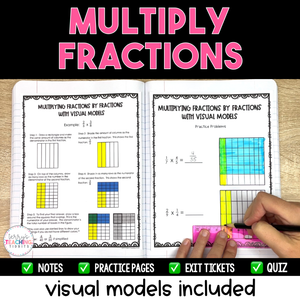# Multiply Fractions Printable Resource Pack - Visual Models Included

• \$12.00
Unit price per

Use visual models and area models along with the standard algorithm to quickly and effectively teach your students how to multiply fractions and multiply mixed numbers using this comprehensive resource pack.

Using visual models and area models, students will have a more hands-on approach to “see” what it looks like when multiplying fractions and mixed numbers in addition to using the standard algorithm.  It’s great for kinesthetic learners as well as students that may struggle with memorizing algorithms.

This resource is complete, concise, and easy to follow with a clear format.  It is low-prep and easy to assign to a sub.  If your students are struggling with fraction operations, this resource is for you!

Standards: 5.NF.4, 5.NF.4a, 5.NF.4b, 5.NF.6

This resource is also part of my 5th Grade Math Year-Long Curriculum Bundle.

How Can You Use This?

Introduce each skill with the detailed set of notes and sample problems.  I suggest doing this in a whole group setting and pulling the PDF up on a Smartboard so students can annotate over the models.

Gradually release students to practice independently using the practice pages.   Check for understanding and mastery after teaching each skill using the exit tickets.

When all skills have been taught, assess with the final quiz when you feel students are ready.

## What You Get

The Mini-Lessons Included:

• Multiply Fractions by Whole Numbers with Visual Models
• Multiply Fractions by Fractions with Visual Models
• Multiply Fractions by Whole Numbers and Fractions Using the Standard Algorithm
• Multiply Mixed Numbers with Area Models
• Multiply Mixed Numbers Using the Standard Algorithm
• Multiply Fractions Word Problems

What You’ll Get in EACH of the mini-lessons:

• Detailed Notes
• Sample Problems
• 1-3 Practice Pages
• 2-3 Exit Tickets
• A couple mini-lessons have Homework Pages

You’ll Also Get:

• Quiz (2 options - with and without area models for multiplying mixed numbers)

## Testimonials

⭐️⭐️⭐️⭐️⭐️ This resource is exactly what I was looking for! It is a unit that is truly built upon Concrete, Representational, and Abstract philosophy. Students learn the "why" of multiplying fractions by using models. This is great for scaffolding instruction.  - BeachTeach5

⭐️⭐️⭐️⭐️⭐️ Wonderful resource for our multiplying fractions unit. Highly engaging! Loved the visual models, practice sheets, and exit slips & quizzes!  - Julie M.

⭐️⭐️⭐️⭐️⭐️ Love these resources!  Easy to use and makes planning so much more efficient.  - Emily B.